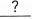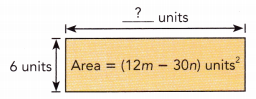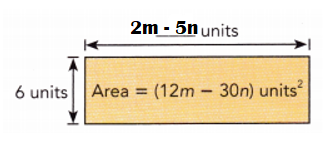# Math in Focus Grade 7 Chapter 3 Lesson 3.5 Answer Key Factoring Algebraic Expressions

Go through the Math in Focus Grade 7 Workbook Answer Key Chapter 3 Lesson 3.5 Factoring Algebraic Expressions to finish your assignments.

## Math in Focus Grade 7 Course 2 A Chapter 3 Lesson 3.5 Answer Key Factoring Algebraic Expressions

### Math in Focus Grade 7 Chapter 3 Lesson 3.5 Guided Practice Answer Key

Copy and complete to factor the expression.
Question 1.
2j – 10 k
2j – 10k =+ ()    Rewrite the expression.
=() +()   The GCF of 2j and -10k is 2.
=Factor 2 from each term.
2j – 10 k = 2 (-5k + j) or 2 (j – 5k).

Explanation:
2j – 10 k = -10k + 2j
= 2 (-5k) + 2 (j)
= 2 (-5k + j) or 2 (j – 5k)

Factor each expression.
Question 2.
6a – 18b
6a – 18b =  3 (2a – 6b).

Explanation:
6a – 18b =  3 (2a) + 3 (-6b)
= 3 (2a – 6b).

Question 3.
8p – 12q
8p – 12q = 4 (2p – 3q).

Explanation:
8p – 12q = 4 (2p) + 4 (-3q)
= 4 (2p – 3q).

Question 4.
-5x – 3
-5x – 3 = -1 (5x + 3).

Explanation:
-5x – 3 = -1 (5x) -1 (3)
= -1 (5x + 3).

Question 5.
-3f – 6
-3f – 6 = -3 (f + 2).

Explanation:
-3f – 6 = -3 (f) – 3 (2)
= -3 (f + 2).

Question 6.
-8p – 10q
-8p – 10q = -2 (4p + 5q).

Explanation:
-8p – 10q = -2 (4p) – 2 (5q)
= -2 (4p + 5q)

### Math in Focus Course 2A Practice 3.5 Answer Key

Factor each expression with two terms.
Question 1.
2x + 8
2x + 8 = 2 (x + 4).

Explanation:
2x + 8 = 2 (x) + 2 (4)
= 2 (x + 4).

Question 2.
5a + 5
5a + 5 = 5 (a + 1).

Explanation:
5a + 5 = 5 (a) + 5 (1)
= 5 (a + 1).

Question 3.
3x – 12
3x – 12 = 3 (x – 4).

Explanation:
3x – 12 = 3 (x) + 3 (-4)
= 3 (x – 4).

Question 4.
4x – 16
4x – 16 = 4 (x – 4).

Explanation:
4x – 16 = 4 (x) + 4 (-4)
= 4 (x – 4).

Question 5.
2x + 8y
2x + 8y = 2 (x + 4y).

Explanation:
2x + 8y = 2 (x) + 2 (4y)
= 2 (x + 4y).

Question 6.
7a + 7b
7a + 7b = 7 (a + b).

Explanation:
7a + 7b = 7 (a) + 7 (b)
= 7 (a + b).

Question 7.
5p + 15q
5p + 15q = 5 (p + 3q).

Explanation:
5p + 15q = 5 (p) + 5 (3q)
= 5 (p + 3q).

Question 8.
14w + 49m
14w + 49m = 7 (2w + 7m).

Explanation:
14w + 49m = 7 (2w) + 7 (7m)
= 7 (2w + 7m).

Question 9.
4j – 16 k
4j – 16 k = 4 (j – 4k).

Explanation:
4j – 16 k = 4(j) + 4(-4k)
= 4 (j – 4k).

Question 10.
8t – 32u
8t – 32u = 8 (t – 4u).

Explanation:
8t – 32u = 8(t) + 8 (-4u)
= 8 (t – 4u).

Question 11.
2a – 10p
2a – 10p = 2 (a – 5p).

Explanation:
2a – 10p = 2 (a) + 2 (-5p)
= 2(a – 5p).

Question 12.
9h – 45f
9h – 45f = 9 (h – 5f).

Explanation:
9h – 45f = 9 (h) + 9 (-5f)
= 9(h – 5f).

Factor each expression with negative terms.
Question 13.
-p – 2
-p – 2 = -1 (p + 2).

Explanation:
-p – 2 = -1 (p) – 1(2)
= -1 (p + 2).

Question 14.
-x – 5
-x – 5 = -1 (x + 5).

Explanation:
-x – 5 = -1 (x) -1 (5)
= -1 (x + 5).

Question 15.
-2d – 7
-2d – 7 = -1 (2d + 7).

Explanation:
-2d – 7 = -1 (2d) – 1(7)
= -1 (2d + 7).

Question 16.
– 4y – 11
– 4y – 11 = -1 (4y + 11).

Explanation:
– 4y – 11 = -1 (4y) – 1(11)
= -1 (4y + 11).

Question 17.
-3a – 6
-3a – 6 = -3 ( a + 2).

Explanation:
-3a – 6 = -3 (a) – 3 (2)
= -3 ( a + 2).

Question 18.
-4x – 20
-4x – 20 = -4 (x + 5).

Explanation:
-4x – 20 = -4(x) – 4 (5)
= -4 (x + 5).

Question 19.
-5k – 25
-5k – 25 = -5 (k + 5).

Explanation:
-5k – 25 = -5 (k) – 5( 5)
= -5 (k + 5).

Question 20.
-7u – 49
-7u – 49 = -7 (u + 7).

Explanation:
-7u – 49 = -7 (u) – 7 (7)
= -7 (u + 7).

Question 21.
-1 – 4n
-1 – 4n = -1 (1 + 4n).

Explanation:
-1 – 4n = -1 (1) – 1(4n)
= -1 (1 + 4n).

Question 22.
-3 – 6a
-3 – 6a = -3 (1 + 2a).

Explanation:
-3 – 6a = -3 (1) – 3( 2a)
= -3 (1 + 2a).

Question 23.
-12x – 16y
-12x – 16y = -4 (3x + 4y).

Explanation:
-12x – 16y = -4 (3x) – 4(4y)
= -4 (3x + 4y).

Question 24.
-25m – 10n
-25m – 10n = -5 (5m + 2n).

Explanation:
-25m – 10n = -5 (5m) – 5(2n)
= -5 (5m + 2n).

Factor each expression with three terms.
Question 25.
4x + 4y + 8
4x + 4y + 8 = 4 (x + y + 2).

Explanation:
4x + 4y + 8 = 4(x) + 4(y) + 4(2)
= 4 (x + y + 2).

Question 26.
2a + 6b + 4
2a + 6b + 4 = 2( a + 3b + 2).

Explanation:
2a + 6b + 4 = 2(a) + 2(3b) + 2(2)
= 2( a + 3b + 2).

Question 27.
5p + 10q + 10
5p + 10q + 10 = 5(p + 2q + 2).

Explanation:
5p + 10q + 10 = 5(p) + 5(2q) + 5(2)
= 5(p + 2q + 2).

Question 28.
12d + 6e + 18
12d + 6e + 18 = 6(2d + e + 3).

Explanation:
12d + 6e + 18 = 6(2d) + 6(e) + 6(3)
= 6(2d + e + 3).

Question 29.
3s – 9t – 15
3s – 9t – 15 = 3(s – 3t – 5).

Explanation:
3s – 9t – 15 = 3(s) + 3(-3t) + 3(-5)
= 3(s – 3t – 5).

Question 30.
4a – 6b – 12
4a – 6b – 12 = 2(2a – 3b – 6).

Explanation:
4a – 6b – 12 = 2(2a) + 2(-3b) + 2(-6)
= 2(2a – 3b – 6).

Question 31.
12a – 9b – 6
12a – 9b – 6 = 3(4a – 3b – 2).

Explanation:
12a – 9b – 6 = 3(4a) + 3(-3b) + 3(-2)
= 3(4a – 3b – 2).

Question 32.
24g – 12h – 36
24g – 12h – 36 = 6(4g – 2h – 6).

Explanation:
24g – 12h – 36 = 6(4g) + 6(-2h) + 6(-6)
= 6(4g – 2h – 6).

Question 33.
A rectangle has an area of (12m – 30n) square units. Its width is 6 units. Factor the expression for the area to find an expression for the length of the rectangle.